Scan QR code or get instant email to install app

Question:

# 1212If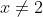and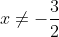, what is the solution to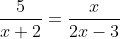?

A 3 and 5
explanation

1212To solve the equation for x, cross multiply to yield x(x + 2) = 5(2x − 3). Simplifying both sides of the new equation results in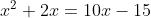. Next, subtract 10x from both sides of the equation and add 15 to both sides of the equation to yield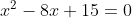. By factoring the left-hand side, the equation can be rewritten in the form (x − 3)(x − 5) = 0. It follows, therefore, that x = 3 and x = 5.